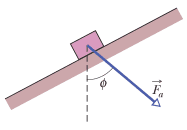# Work done by an applied force

nothingsus

## Homework Statement

A constant force F of magnitude 82.0 N is applied to a 3.00 kg shoe box at angle phi = 53.0°, causing the box to move up a frictionless ramp at constant speed. How much work is done on the box by when the box has moved through vertical distance h = 0.150 m?W=Fdcos(theta)

## The Attempt at a Solution

So I understand that you can use net work = W_g + W_f = change in KE = 0 (since constant speed) to solve this so W_f = mgd = 3*9.8*0.150 = , but I'm curious why you can't just use the work equation.

Here's my reasoning. Please explain where I'm incorrect.

The applied force on the box acts at 53 degrees to the vertical and causes a displacement of 0.150m straight up. The angle between the force vector and the displacement vector is 180-53 = 127 degrees. so to calculate the work done on the box by the applied force only

W = 82*0.150*cos(127) = -7.40 J

It's negative (and also the wrong magnitude) which doesn't make sense because energy is supposed to be transferred to the box due to the force to move it upwards...?

Why can't I just use the work equation to find work done by a specific force only (the applied force in this case) like I have in other questions?

WrongMan
well i'd say that its because de box didn't move straight up.
It'd makes sense that if the box were moving straight up the work done by the force would be negative, since its opposing the box movement.

You cant use the work equation because you dont know the actual displacement of the box, the triangle with height 0.15 can have an infinite number of sides attached to it

Furthermore, you do the cosine of the angle, to find the force's component in the direction that the box moved. looking at the picture, the y component of the force in the y direction is downward (thats what you calculated~when doing Fcos(127)), the box doesnt move up due to the y component of the force, it moves up due to the x component.

Edit: to use the work formula here's what you'd have to do:
Find the component of F along the inclined surface, then find the component of that force with the vertical, and that should work...

Homework Helper
Gold Member
causing the box to move up a frictionless ramp at constant speed
What does this tell you about the net force on the box?
Edit: I see you have used W=mgh and I think my question is of no use. I believe since the angle of incline is unknown, you can't determine the angle between force and displacement.

Mentor
Let ##\theta## be the angle that the ramp makes with the horizontal. In terms of ##\phi## and ##\theta##, what are the components of the force F in the directions parallel and perpendicular to the ramp? Considering that the net force on the box is zero in the parallel direction, and the component of weight in the parallel direction is ##-mg\sin \theta##, what is the force balance on the box in the parallel direction?